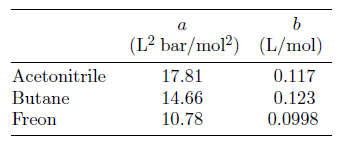# Problem: Which gas molecule do you expect to be the largest? (a and b are Van der Waals constants.) 1. Butane 2. Acetonitrile 3. Freon

###### FREE Expert Solution
84% (395 ratings)
###### Problem Details

Which gas molecule do you expect to be the largest? (a and b are Van der Waals constants.)

1. Butane

2. Acetonitrile

3. FreonWhat scientific concept do you need to know in order to solve this problem?

Our tutors have indicated that to solve this problem you will need to apply the Van der Waals Equation concept. You can view video lessons to learn Van der Waals Equation. Or if you need more Van der Waals Equation practice, you can also practice Van der Waals Equation practice problems.

What is the difficulty of this problem?

Our tutors rated the difficulty ofWhich gas molecule do you expect to be the largest? (a and b...as medium difficulty.

How long does this problem take to solve?

Our expert Chemistry tutor, Dasha took undefined 40 seconds to solve this problem. You can follow their steps in the video explanation above.

What professor is this problem relevant for?

Based on our data, we think this problem is relevant for Professor Bucks' class at UC.